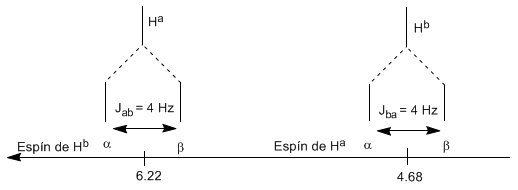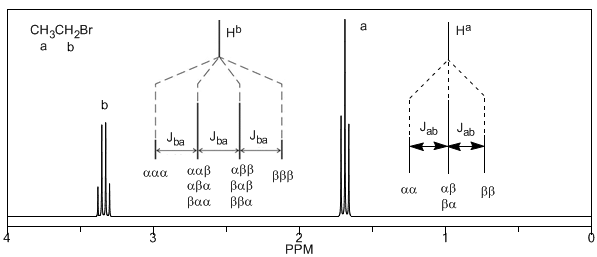The structural information of NMR derives from two factors: the different displacements observed depending on the chemical environment that surrounds the proton and the coupling between the spins of nearby protons, which produces the splitting of the signals.

Although some signals in the spectrum are single peaks, it is common to find signals composed of several very close peaks, which are named with the following notation: singlet (s), doublet (d), triplet (t), quadruplet (c), quintuplet ( q), sextuplet (sx) and septuplet (sp), complex signals are designated as multiplets. The value of $\delta$ of these signals is assigned to their center, unless the multiplet is irregular, in which case the interval is indicated.In the spectrum of ethanol it can be seen that the hydroxyl hydrogen produces a singlet, the pair of hydrogens on carbon one give rise to a quadruplet, and the three hydrogens on carbon two produce a triplet.

Explanation of spin-spin coupling.

To understand the splitting of signals due to spin-spin coupling we are going to study the spectrum of 1,1-dichloro-2,2-diphenylethane ($Cl_2CH^{a}CH^{b}Ph_2$).The proton $H^a$ subjected to a magnetic field $B_0$ produces a signal at $\delta_a=6.22 ppm$. However, the proton $H_b$ generates a small magnetic field that affects the proton $H_a$. Approximately half of the molecules have the $H_b$ proton aligned with the applied field (alpha spin) and the other half have it oriented against the field (beta spin). When $H_b$ has spin $\alpha$, $H_a$ is subjected to a slightly larger field and resonates at a higher frequency ($\delta$ slightly higher). When $H_b$ has spin $\beta$, $H_a$ is subjected to a slightly smaller field and resonates at a lower frequency ($\delta$ slightly lower), which produces the splitting of the initial peak into two signals separated by a distance of 4 Hz, called the coupling constant (J). This same reasoning can be done for the proton $H_b$.Next, we will study the coupling of a hydrogen $H^a$ that has two neighboring hydrogens $H^b$. In this situation, the splitting of the hydrogen signal $H^a$ into three peaks (triplet) is observed, the central peak being twice as intense as the extreme ones. For their part, the hydrogens $H^b$ couple with $H^a$ producing two peaks of equal intensity (doublet).Lastly, we will discuss the coupling of a proton with three equivalent neighboring protons. In this case, a signal made up of four peaks (quadtriplet) is observed. The central peaks are three times as intense as the extreme peaks.